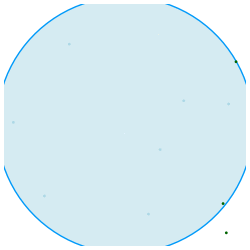$\newcommand{\eps}{\varepsilon} \newcommand{\kron}{\otimes} \DeclareMathOperator{\diag}{diag} \DeclareMathOperator{\trace}{trace} \DeclareMathOperator{\rank}{rank} \DeclareMathOperator*{\minimize}{minimize} \DeclareMathOperator*{\maximize}{maximize} \DeclareMathOperator{\subjectto}{subject to} \newcommand{\mat}{\boldsymbol{#1}} \renewcommand{\vec}{\boldsymbol{\mathrm{#1}}} \newcommand{\vecalt}{\boldsymbol{#1}} \newcommand{\conj}{\overline{#1}} \newcommand{\normof}{\|#1\|} \newcommand{\onormof}{\|#1\|_{#2}} \newcommand{\MIN}{\begin{array}{ll} \minimize_{#1} & {#2} \end{array}} \newcommand{\MINone}{\begin{array}{ll} \minimize_{#1} & {#2} \\ \subjectto & {#3} \end{array}} \newcommand{\MINthree}{\begin{array}{ll} \minimize_{#1} & {#2} \\ \subjectto & {#3} \\ & {#4} \\ & {#5} \end{array}} \newcommand{\MAX}{\begin{array}{ll} \maximize_{#1} & {#2} \end{array}} \newcommand{\MAXone}{\begin{array}{ll} \maximize_{#1} & {#2} \\ \subjectto & {#3} \end{array}} \newcommand{\itr}{#1^{(#2)}} \newcommand{\itn}{^{(#1)}} \newcommand{\prob}{\mathbb{P}} \newcommand{\probof}{\prob\left\{ #1 \right\}} \newcommand{\pmat}{\begin{pmatrix} #1 \end{pmatrix}} \newcommand{\bmat}{\begin{bmatrix} #1 \end{bmatrix}} \newcommand{\spmat}{\left(\begin{smallmatrix} #1 \end{smallmatrix}\right)} \newcommand{\sbmat}{\left[\begin{smallmatrix} #1 \end{smallmatrix}\right]} \newcommand{\RR}{\mathbb{R}} \newcommand{\CC}{\mathbb{C}} \newcommand{\eye}{\mat{I}} \newcommand{\mA}{\mat{A}} \newcommand{\mB}{\mat{B}} \newcommand{\mC}{\mat{C}} \newcommand{\mD}{\mat{D}} \newcommand{\mE}{\mat{E}} \newcommand{\mF}{\mat{F}} \newcommand{\mG}{\mat{G}} \newcommand{\mH}{\mat{H}} \newcommand{\mI}{\mat{I}} \newcommand{\mJ}{\mat{J}} \newcommand{\mK}{\mat{K}} \newcommand{\mL}{\mat{L}} \newcommand{\mM}{\mat{M}} \newcommand{\mN}{\mat{N}} \newcommand{\mO}{\mat{O}} \newcommand{\mP}{\mat{P}} \newcommand{\mQ}{\mat{Q}} \newcommand{\mR}{\mat{R}} \newcommand{\mS}{\mat{S}} \newcommand{\mT}{\mat{T}} \newcommand{\mU}{\mat{U}} \newcommand{\mV}{\mat{V}} \newcommand{\mW}{\mat{W}} \newcommand{\mX}{\mat{X}} \newcommand{\mY}{\mat{Y}} \newcommand{\mZ}{\mat{Z}} \newcommand{\mLambda}{\mat{\Lambda}} \newcommand{\mPbar}{\bar{\mP}} \newcommand{\ones}{\vec{e}} \newcommand{\va}{\vec{a}} \newcommand{\vb}{\vec{b}} \newcommand{\vc}{\vec{c}} \newcommand{\vd}{\vec{d}} \newcommand{\ve}{\vec{e}} \newcommand{\vf}{\vec{f}} \newcommand{\vg}{\vec{g}} \newcommand{\vh}{\vec{h}} \newcommand{\vi}{\vec{i}} \newcommand{\vj}{\vec{j}} \newcommand{\vk}{\vec{k}} \newcommand{\vl}{\vec{l}} \newcommand{\vm}{\vec{l}} \newcommand{\vn}{\vec{n}} \newcommand{\vo}{\vec{o}} \newcommand{\vp}{\vec{p}} \newcommand{\vq}{\vec{q}} \newcommand{\vr}{\vec{r}} \newcommand{\vs}{\vec{s}} \newcommand{\vt}{\vec{t}} \newcommand{\vu}{\vec{u}} \newcommand{\vv}{\vec{v}} \newcommand{\vw}{\vec{w}} \newcommand{\vx}{\vec{x}} \newcommand{\vy}{\vec{y}} \newcommand{\vz}{\vec{z}} \newcommand{\vpi}{\vecalt{\pi}} \newcommand{\vlambda}{\vecalt{\lambda}}$# Numerical analysis

## Announcements

2016-11-30
The optional extra credit homework is due Thursday 2016-12-15 by 3pm.
2016-11-13
Please complete the Homework 6 by 5pm on 2016-11-22
2016-10-28
Please complete the Homework 5 by 5pm on 2016-11-07
2016-10-06
Please complete the Homework 4 by Midnight on 2016-10-14
2016-09-25
Please complete the Homework 3 by Midnight on 2016-09-30
2016-09-08
Please complete the Homework 2 by 5pm on 2016-09-16
2016-08-24
Please complete the Homework 1 by class on 2016-09-02
2016-08-22
Please complete the intro survey by class on 2016-08-27

## Overview

Numerical methods is a class that will introduce you to one of the ways that computers were first used: to solve problems and equations arising from mathematics and physics. It will also feature modern topics such as web-ranking algorithms and how they are all tied together via a set of numerical computing primitives. This class will study how to make useful and meaningful approximations of numbers that would take infinite computer memory to represent exactly, and how to do these things quickly. We'll cover these topics in three units

### Unit 1: Mathematical modeling, numerical programming, Monte Carlo, and floating point

• How to turn a verbal problem description into a model we can use the computer to solve
• How to use the Matlab programming language and environment
• How computers represent numbers like (1/3) and floating point arithmetic
• How randomized and Monte Carlo methods let us approximate complicated mathematical quantites quickly.
• Topics round-off analysis, numerically stable method, mathematical model, Matlab

### Unit 2: Matrix methods

• Understand why matrix multiplication is so important and how to implement it.
• How to solve linear systems of equations using direct and iterative methods
• Conditioning, or how the problem affects how hard it is to solve.
• How to find eigenvalues of matrices
• Topics Gaussian elimination, GEMM, BLAS, LAPACK, condition number, Power method, eigenvalues, Gauss-Seidel, Conjugate Gradients

### Unit 3: Applied mathematics

• Types of approximations and their accuracy
• Why we need interpolation and how to interpolate
• How to get the computer to integrate a function accurately
• How to get the computer to approximate a derivative
• How to solve an ordinary differential equation and a partial differential
• An introduction to numerical optimization and solving nonlinear systems (Newton's method, bisection search)
• Topics Quadrature, Chebfun, Euler methods, Accuracy and Stability, Newton's method, Gradient Descent, Line search, Root finding, Golden section search, Gaussian quadrature,

### Logistics

Homeworks will be due on Blackboard and we'll have class discussions on Piazza

## Prerequisties

See the course catalog for the latest prerequisites.